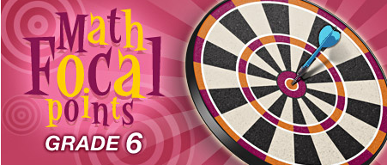### Introduction

In an effort to highlight the most important mathematical topics at each grade level, the National Council of Teachers of Mathematics (NCTM) has developed Curriculum Focal Points for Prekindergarten Through Grade 8 Mathematics. This second in a series of Middle School Portal publications supports the teaching of the topics selected for grade 6. The first in the series featured teaching of the focal points for grade 5.

NCTM emphasizes that a “focal point” is a “cluster of related knowledge, skills, and concepts,” rather than a discrete topic to be checked off a list. The focal points, three at each grade level, specify “the mathematical content that a student needs to understand deeply and thoroughly for future mathematics learning.”

In the sections of this publication we offer resources on the three areas of emphasis highlighted for sixth grade. (For a complete statement of the NCTM Curriculum Focal Points for grade 6, please see below.)

NCTM recommends that students at the sixth-grade level work on developing an understanding and mastery of multiplication and division of fractions and decimals.. In the section titled Fractions and Decimals, you will find visual and interactive explanations of the difficult concepts underpinning multiplication and division of fractions and decimals, plus opportunities for your students to practice their skills.

Students at this level should also be connecting ratio and rate to multiplication and division. The games, problems, and lesson ideas in Ratio and Rate are intended to help students extend their understanding of equivalent fractions to ratio and rate and their mastery of whole number multiplication and division to solve problems that use these concepts.

In grade 6, students should also be writing, interpreting, and using mathematical expressions and equations. The resources in Expressions and Equations focus on work with variables and simple equations: writing and evaluating expressions, solving one-step equations, or applying algebraic thinking to problems.

In Background Information for Teachers, you will find professional learning resources. Finally, in NCTM Standards we discuss the focal points as they are related to the Principles and Standards for School Mathematics.

We hope the resources in this and the other publications in this series open your classes to a wider view of mathematics!

#### NCTM Curriculum Focal Points for Grade 6

Number and Operations: Developing an understanding of and fluency with multiplication and division of fractions and decimals. Students use the meanings of fractions, multiplication and division, and the inverse relationship between multiplication and division to make sense of procedures for multiplying and dividing fractions and explain why they work. They use the relationship between decimals and fractions, as well as the relationship between finite decimals and whole numbers (i.e., a finite decimal multiplied by an appropriate power of 10 is a whole number), to understand and explain the procedures for multiplying and dividing decimals. Students use common procedures to multiply and divide fractions and decimals efficiently and accurately. They multiply and divide fractions and decimals to solve problems, including multistep problems and problems involving measurement.

Number and Operations: Connecting ratio and rate to multiplication and division. Students use simple reasoning about multiplication and division to solve ratio and rate problems (e.g., “If 5 items cost \$3.75 and all items are the same price, then I can find the cost of 12 items by first dividing \$3.75 by 5 to find out how much one item costs and then multiplying the cost of a single item by 12”). By viewing equivalent ratios and rates as deriving from, and extending, pairs of rows (or columns) in the multiplication table, and by analyzing simple drawings that indicate the relative sizes of quantities, students extend whole number multiplication and division to ratios and rates. Thus, they expand the repertoire of problems that they can solve by using multiplication and division, and they build on their understanding of fractions to understand ratios. Students solve a wide variety of problems involving ratios and rates.

Algebra: Writing, interpreting, and using mathematical expressions and equations. Students write mathematical expressions and equations that correspond to given situations, they evaluate expressions, and they use expressions and formulas to solve problems. They understand that variables represent numbers whose exact values are not yet specified, and they use variables appropriately. Students understand that expressions in different forms can be equivalent, and they can rewrite an expression to represent a quantity in a different way (e.g., to make it more compact or to feature different information). Students know that the solutions of an equation are the values of the variables that make the equation true. They solve simple one-step equations by using number sense, properties of operations, and the idea of maintaining equality on both sides of an equation. They construct and analyze tables (e.g., to show quantities that are in equivalent ratios), and they use equations to describe simple relationships (such as 3x = y) shown in a table.

Reprinted with permission from Curriculum Focal Points for Prekindergarten through Grade 8 Mathematics: A Quest for Coherence, copyright 2006 by the National Council of Teachers of Mathematics. All rights reserved.

Non-profit Tax ID # 203478467